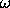## 3.11  Projects

Exercise 3.14.  Periodically driven pendulum
Explore the dynamics of the driven pendulum, using the surface of section method. We are interested in exploring the regions of parameter space over which various phenomena occur. Consider a pendulum of length 9.8 m, mass 1 kg, and acceleration of gravity g = 9.8 m s-2, giving0 = 1 rad s-1. Explore the parameter plane of the amplitude A and frequencyof the periodic drive.

Examples of the phenomena to be investigated:

a.  Inverted equilibrium. Show the region of parameter space (A,) in which the inverted equilibrium is stable. If the inverted equilibrium is stable there is some range of stability, i.e., there is a maximum angle of displacement from the equilibrium that stable oscillations reach. If you have enough time, plot contours in the parameter space for different amplitudes of the stable region.

b.  Period doubling of the normal equilibrium. For this case, plot the angular momenta of the stable and unstable equilibria as functions of the frequency for some given amplitude.

c.  Transition to large-scale chaos. Show the region of parameter space (A,) for which the chaotic zones around the three principal resonance islands are linked.

Exercise 3.15.  Spin-orbit surfaces of section
Write a program to compute surfaces of section for the spin-orbit problem, with the section points being recorded at pericenter. Investigate the following:

a.  Give a Hamiltonian formulation of the spin-orbit problem introduced in section 2.11.2.

b.  For out-of-roundness parameter= 0.1 and eccentricity e = 0.1, measure the widths of the regular islands associated with the 1:1, 3:2, and 1:2 resonances.

c.  Explore the surfaces of section for a range offor fixed e = 0.1. Estimate the critical value ofabove which the main chaotic zones around the 3:2 and the 1:1 resonance islands are merged.

d.  For a fixed eccentricity e = 0.1 trace the location on the surface of section of the stable and unstable fixed points associated with the 1:1 resonance as a function of the out-of-roundness.